Chin. Phys. B, 2020, Vol. 29(11): 116805    DOI: 10.1088/1674-1056/abc0d5
Special Issue: SPECIAL TOPIC — Machine learning in condensed matter physics
 Prev   Next

# Machine learning identification of impurities in the STM images

Ce Wang(王策)1, Haiwei Li(李海威)2, Zhenqi Hao(郝镇齐)2, Xintong Li(李昕彤)2, Changwei Zou(邹昌炜)2, Peng Cai(蔡鹏)3, †, Yayu Wang(王亚愚)2,4, Yi-Zhuang You(尤亦庄)5,, ‡, and Hui Zhai(翟荟)1,§
1 Institute for Advanced Study, Tsinghua University, Beijing 100084, China
2 State Key Laboratory of Low Dimensional Quantum Physics, Department of Physics, Tsinghua University, Beijing 100084, China
3 Department of Physics and Beijing Key Laboratory of Opto-electronic Functional Materials and Micro-nano Devices, Renmin University of China, Beijing 100872, China
4 Frontier Science Center for Quantum Information, Beijing 100084, China
5 Department of Physics, University of California, San Diego, California 92093, USA
 Abstract  We train a neural network to identify impurities in the experimental images obtained by the scanning tunneling microscope (STM) measurements. The neural network is first trained with a large number of simulated data and then the trained neural network is applied to identify a set of experimental images taken at different voltages. We use the convolutional neural network to extract features from the images and also implement the attention mechanism to capture the correlations between images taken at different voltages. We note that the simulated data can capture the universal Friedel oscillation but cannot properly describe the non-universal physics short-range physics nearby an impurity, as well as noises in the experimental data. And we emphasize that the key of this approach is to properly deal with these differences between simulated data and experimental data. Here we show that even by including uncorrelated white noises in the simulated data, the performance of the neural network on experimental data can be significantly improved. To prevent the neural network from learning unphysical short-range physics, we also develop another method to evaluate the confidence of the neural network prediction on experimental data and to add this confidence measure into the loss function. We show that adding such an extra loss function can also improve the performance on experimental data. Our research can inspire future similar applications of machine learning on experimental data analysis. Keywords:  scanning tunneling microscope      neural network      attention      data regularization Received:  02 July 2020      Revised:  07 August 2020      Accepted manuscript online:  14 October 2020 Fund: HZ is supported by Beijing Outstanding Scholar Program, the National Key Research and Development Program of China (Grant No. 2016YFA0301600), and the National Natural Science Foundation of China (Grant No. 11734010). YZY is supported by a startup fund from UCSD. PC is supported by the Fundamental Research Funds for the Central Universities, and the Research Funds of Renmin University of China. Corresponding Authors:  †Corresponding author. E-mail: pcai@ruc.edu.cn ‡Corresponding author. E-mail: yzyou@ucsd.edu §Corresponding author. E-mail:hzhai@tsinghua.edu.cn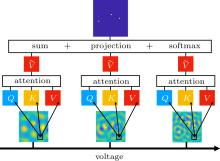Fig. 1.  The structure of the NN. The voltage arrow indicates a sequence of images taken at different voltages. Each image ${X}_{i}^{l}$ is mapped to tensors of the query ${Q}_{i}^{l}$, the key ${K}_{i}^{l}$, and the value ${V}_{i}^{l}$. By the attention mechanism, it leads to normalized value ${\tilde{V}}_{i}^{l}$ computed by Eq. (1). Then, we sum over all ${\tilde{V}}_{i}^{l}$ and reach the output following a linear projection and a Softmax.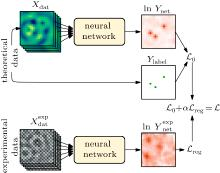Fig. 2.  Computation graph for the loss function. The NN is trained on the theoretical data via the regression loss ${ {\mathcal L} }_{0}$, and regularized by the experimental data via the prediction confidence regularization ${ {\mathcal L} }_{{\rm{reg}}}$.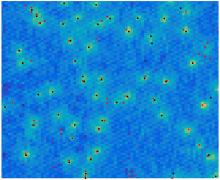Fig. 3.  Comparison between the prediction of the NN and a reference answer from another experiment. Red squares are the prediction of NN, determined by local maxima of the ${\mathscr{S}}$ layer generated by the NN. Black dots are the reference answer from another experiment, determined by the local maxima of the DoS of bound states shown as the color plot. Here, for training the NN, we use loss function ${ {\mathcal L} }_{0}+\alpha { {\mathcal L} }_{{\rm{reg}}}$ with α = 0.03 and training data with η = 0.8 Gaussian noise.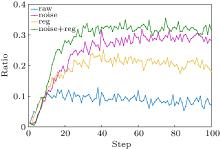Fig. 4.  Compare the performance of different approaches on the experimental data. The curves are the ratio of the reference points perfectly agreed with prediction to the total reference points. The blue line and the purple line are given by NN trained by loss function ${ {\mathcal L} }_{0}$, and the training data have no noise for the blue line and include noise for the purple line. The yellow line is given by NN trained by loss function ${ {\mathcal L} }_{0}+\alpha { {\mathcal L} }_{{\rm{reg}}}$ including confidence regularization and data without noise. Finally, the green line is given by NN trained by loss function ${ {\mathcal L} }_{0}+\alpha { {\mathcal L} }_{{\rm{reg}}}$ and data with noise. Here we take α = 0.03. The ratios are plotted as a function of the training epoch, and the results are averaged over ten independent training processes.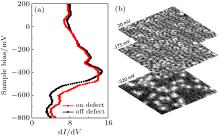Fig. A1.  Experimental data on Au(111) surface. (a) Typical dI/dV spectra measured on defect (red curve) and on defect-free area (black curve). The abrupt increase of spectra above −520 mV shows the itinerant surface states on Au(111). Additional spectra on black curve indicates the bound state of defect below the surface band. (b) Differential conductance maps. Top panel, two representative dI/dV maps (at −20 mV and −175 mV) taken at 5 K with tunneling junction R = 5 GΩ, showing spatial oscillation from the quantum interference of surface states. Bottom, the conductance map at −520 mV, revealing the spatial distribution of the defect states.